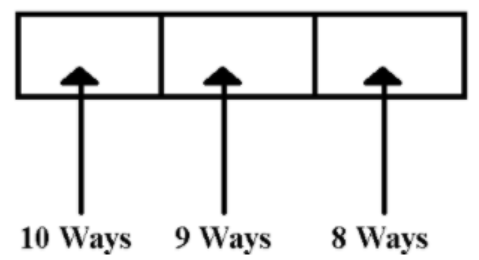A number lock on a suitcase has 3 wheels each labelled with 10 digits from 0 to 9. If the opening of the lock is a particular sequence of three digits with no repeats, how many such sequences will be possible?Verified
146.1k+ views
Hint: As, it is given in the equation that there is no repetition allowed therefore all the three wheels will have different different numbers. On the first wheel there will be 10 ways of selecting numbers, after that the other two will have 9 numbers and 8 numbers to be selected.

Complete step by step solution: As, the number lock has three wheels and there is no repetition allowed.
So the ways of selecting the numbers will be as follows,
On the first wheel from 0 to 9 any number can be put up, therefore the number of ways of selecting will be 10 ways. On the second wheel from 0 to 9, only 9 numbers can be selected, as there is no repetition allowed because on the first wheel one number has already been put up, so there will be 9 numbers in hand to put up on the second wheel. On the third wheel from 0 to 9, only 8 numbers can be selected, as there is no repetition allowed because on the first wheel one number has already been put up and on the second wheel another number has been put up, so there will be 8 numbers in hand to put up on the third wheel.Therefore, the total number of ways of selecting or the total number of sequences possible will be equal to the product of ways on number that can be put on the first, second and third wheel, i.e., $10 \times 9 \times 8$ which is equal to 720.

Note: This question involves the concept of combination, the basic definition of combination is the way of arranging and selecting r number of objects out n objects and it is denoted by $^n{C_r}$.
This question can be solved also using the formula of combination, on the first wheel we need to select one number out ten numbers, i.e., $^{10}{C_1}$, similarly for the second wheel number of ways the selecting will be $^9{C_1}$, because repetition is not allowed and for the third wheel only eight numbers are left to select. i.e., $^8{C_1}$.
So, the total number of selecting will be $^{10}{C_1}{ \times ^9}{C_1}{ \times ^8}{C_1} = 10 \times 9 \times 8 = 720$.